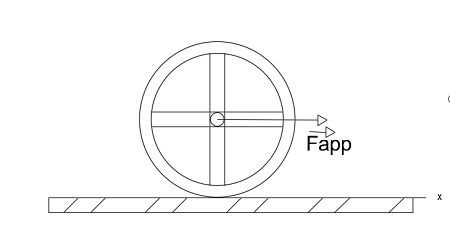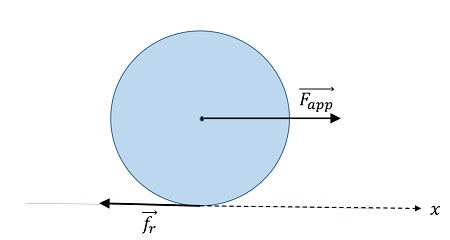# In the figure, a constant horizontal force \vec{F_{app}} of magnitude 15 N is applied to a wheel...

## Question:

In the figure, a constant horizontal force {eq}\displaystyle \vec{F_{app}} {/eq} of magnitude {eq}\displaystyle 15 \ N {/eq} is applied to a wheel of mass {eq}\displaystyle 6.6 \ kg {/eq} and radius {eq}\displaystyle 0.51 \ m {/eq}. The wheel rolls smoothly on the horizontal surface, and the acceleration of its center of mass has magnitude {eq}\displaystyle 1.3 \ m/s^2 {/eq}.

A.) What is the magnitude of the frictional force on the wheel?

B.) What is the rotational inertia of the wheel about the rotation axis through its center of mass?## Rotation into linear movement

A circular body is said to roll smoothly on a surface when the rotation movement completely transforms into a linear movement. In this case the rotated angle is related to the displacement of the body in accordance to,

{eq}\Delta x = R \Delta \theta {/eq},

where {eq}R {/eq} is the radius of the circular body, {eq}\Delta x {/eq} is the linear displacement and {eq}\Delta \theta {/eq} is the rotated angle.

This constraint relates also the linear and angular velocities and accelerations ,

{eq}v=R\omega {/eq},

and,

{eq}a=R\alpha {/eq}.

This type of movement is associated with the frictional forces between the body and the surface and their capacity to totally transform the rotation into linear displacement. If the rotation is to fast or the linear velocity to big for the friction to respond the body will slid or continuously rotate without moving. Both situations can be seen in cars, the first one for a sudden break and the second for an over accelerated start.

## Answer and Explanation:

The wheel moves along the positive {eq}x {/eq} direction under the action of the external force and the friction as shown in the diagram bellow,The Newton equations for the linear and rotation movement are simply,

{eq}\vec{F}_{app}+\vec{f}_r=M\vec{a} {/eq},

the vector notation can be immediately dropped as all the force lay on the {eq}x {/eq} axis,

{eq}F_{app}-f_r=Ma \qquad\qquad(1) {/eq}.

For the rotation movement we get,

{eq}\vec{\tau}_{f_r}=I\vec{\alpha} {/eq},

the only force doinng torque is the friction as {eq}\vec{F}_{app} {/eq} acts on the axis of rotation of the wheel. In scalar form the equation takes the form,

{eq}\tau_{f_r}=I\alpha {/eq},

the torque propels the wheel to rotate in the clockwise direction.

The torque of the friction can be calculated as,

{eq}\tau_{f_r}=Rf_r\sin\gamma {/eq},

with {eq}\gamma {/eq} being the angle formed between the force and the radius. In this case {eq}\gamma=\pi/2 {/eq} as the friction is tangential to the circumference. Hence,

{eq}\tau_{f_r}=Rf_r {/eq},

substituting into Newton's law for rotation we get,

{eq}Rf_r=I\alpha \qquad\qquad (2) {/eq}.

To solve for the friction and the moment of inertia we use equations (1) and (2) with the constraint mentioned in the context for the accelerations of a smoothly rolling wheel.

{eq}\alpha=\dfrac{a}{R} {/eq}.

A) As {eq}a {/eq} is known the friction is simply,

{eq}f_r=F_{app}-Ma=6.42 {/eq} N.

B) Next for the moment of inertia {eq}I {/eq} we use equation (2),

{eq}I=\dfrac{Rf_r}{\alpha}=\dfrac{R^2f_r}{a}=1.28 {/eq} kgm{eq}^2 {/eq}.

#### Learn more about this topic:Practice Applying Newton's Second Law

from Physics 111: Physics I

Chapter 6 / Lesson 9
2.5K